## Pythagorean Theorem and Its Converse

In Figure 1CD is the altitude to hypotenuse AB.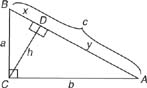Figure 1 An altitude drawn to the hypotenuse of a right triangle to aid in deriving the Pythagorean theorem.

So, by Theorem 63,

• ca = ax, which becomes a2 = cx
• and cb = by, which becomes b2 = cy

From the addition property of equations in algebra, we get the following equation.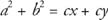By factoring out the c on the right side,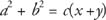But x + y = c (Segment Addition Postulate),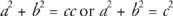This result is known as the Pythagorean Theorem.

Theorem 65 (Pythagorean Theorem): In any right triangle, the sum of the squares of the legs equals the square of the hypotenuse (leg2 + leg2 = hypotenuse2). See Figure 2 for the parts of a right triangle.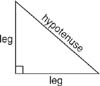Figure 2 Parts of a right triangle.

Example 1: In Figure 3, find x, the length of the hypotenuse.Figure 3 Using the Pythagorean Theorem to find the hypotenuse of a right triangle.Example 2: Use Figure 4 to find x.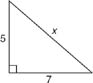Figure 4 Using the Pythagorean Theorem to find the hypotenuse of a right triangle.Any three natural numbers, a, b, c, that make the sentence a2 + b2 = c2 true are called a Pythagorean triple. Therefore, 3‐4‐5 is called a Pythagorean triple. Some other values for ab, and c that will work are 5‐12‐13 and 8‐15‐17. Any multiple of one of these triples will also work. For example, using the 3‐4‐5: 6‐8‐10, 9‐12‐15, and 15‐20‐25 are also Pythagorean triples.

Example 3: Use Figure 5 to find x.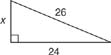Figure 5 Using the Pythagorean Theorem to find a leg of a right triangle.

If you can recognize that the numbers x, 24, 26 are a multiple of the 5‐12‐13 Pythagorean triple, the answer for x is quickly found. Because 24 = 2(12) and 26 = 2(13), then x = 2(5) or x = 10. You can also find x by using the Pythagorean Theorem.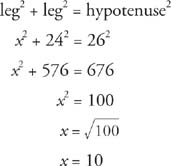Example 4: Use Figure 6 to find x.Figure 6 Using the Pythagorean Theorem to find the unknown parts of a right triangle.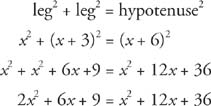Subtract x2 + 12 x + 36 from both sides.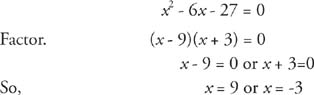But x is a length, so it cannot be negative. Therefore, x = 9.

The converse (reverse) of the Pythagorean Theorem is also true.

Theorem 66: If a triangle has sides of lengths a, b, and c where c is the longest length and c2 = a2 + b2, then the triangle is a right triangle with c its hypotenuse.

Example 5: Determine if the following sets of lengths could be the sides of a right triangle: (a) 6‐5‐4, (b)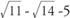, (c) 3/4‐1‐5/4.

(a) Because 6 is the longest length, do the following check.So 4‐5‐6 are not the sides of a right triangle.

(b) Because 5 is the longest length, do the following check.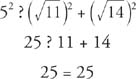Soare sides of a right triangle, and 5 is the length of the hypotenuse.

(c) Because 5/4 is the longest length, do the following check.So 3/4‐1‐5/4 are sides of a right triangle, and 5/4 is the length of the hypotenuse.